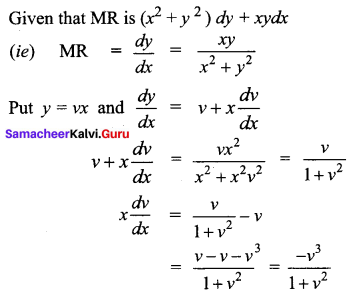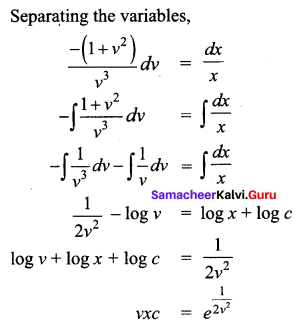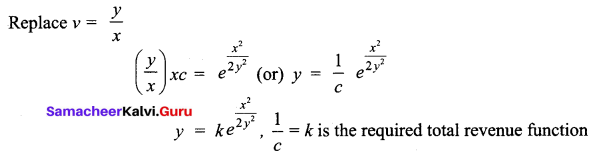Students can download 12th Business Maths Chapter 4 Differential Equations Ex 4.3 Questions and Answers, Samacheer Kalvi 12th Business Maths Book Solutions Guide Pdf helps you to revise the complete Tamilnadu State Board New Syllabus and score more marks in your examinations.

## Tamilnadu Samacheer Kalvi 12th Business Maths Solutions Chapter 4 Differential Equations Ex 4.3

Solve the following homogeneous differential equations

Question 1.
x $$\frac{d y}{d x}$$ = x + y
Solution: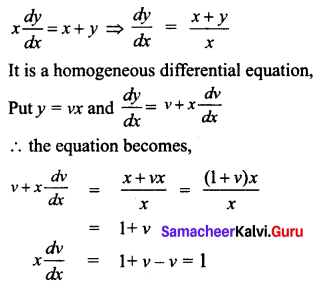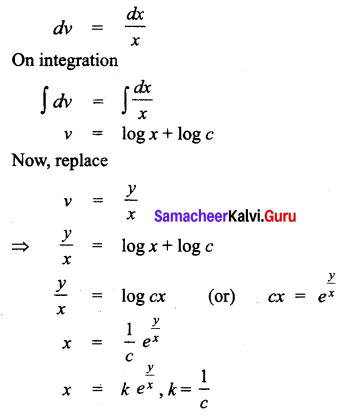Question 2.
(x – y) $$\frac{d y}{d x}$$ = x + 3y
Solution: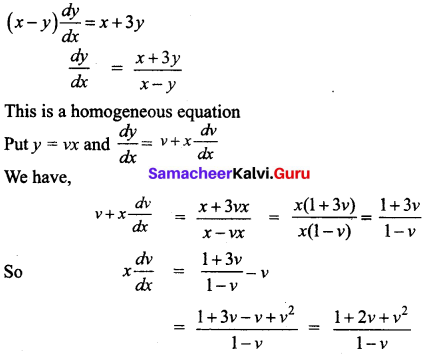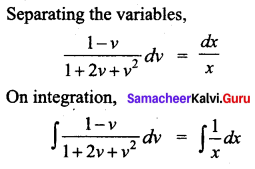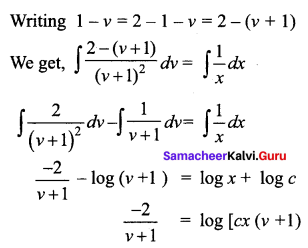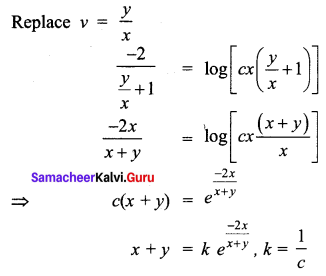Question 3.
$$x \frac{d y}{d x}-y=\sqrt{x^{2}+y^{2}}$$
Solution: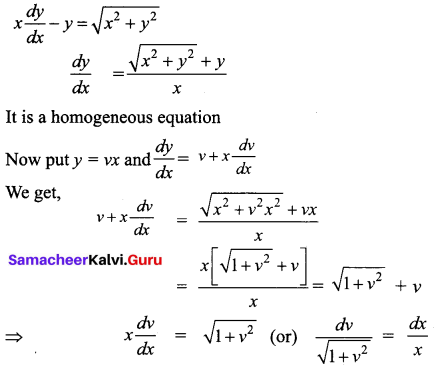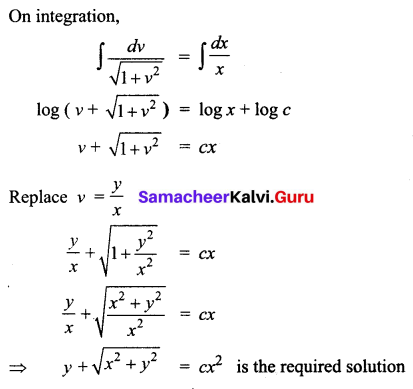Question 4.
$$\frac{d y}{d x}=\frac{3 x-2 y}{2 x-3 y}$$
Solution: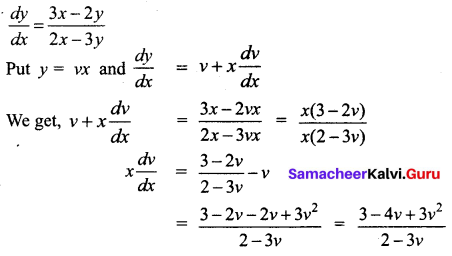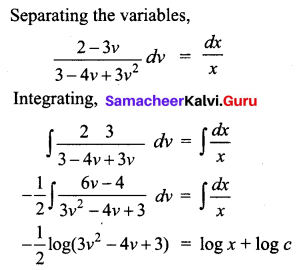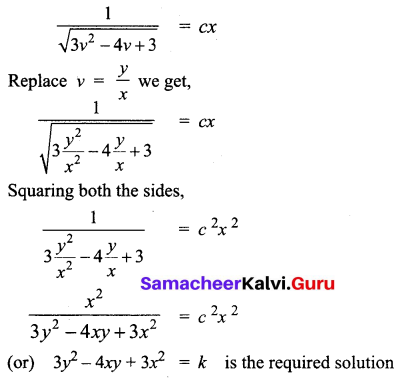Question 5.
(y2 – 2xy) dx = (x2 – 2xy) dy
Solution: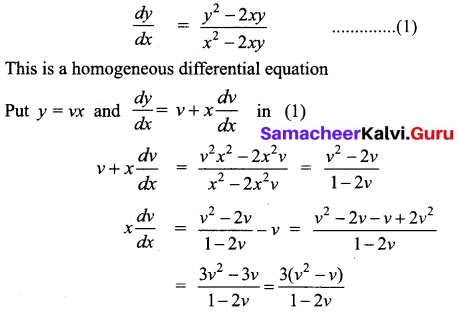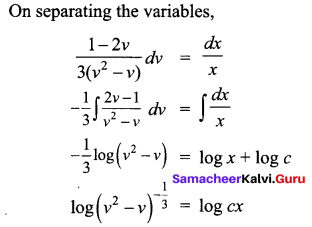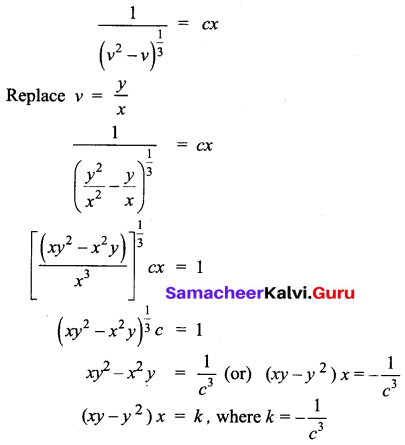Question 6.
The slope of the tangent to a curve at any point (x, y) on it is given by (y3 – 2yx2) dx + (2xy2 – x3) dy = 0 and the curve passes through (1, 2). Find the equation of the curve.
Solution: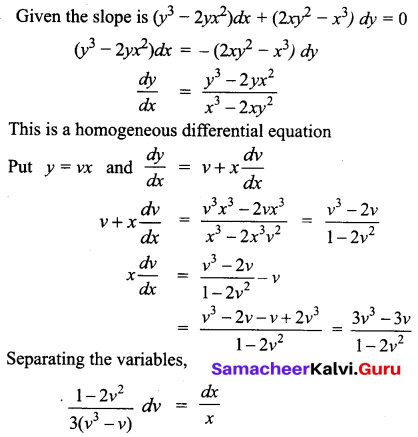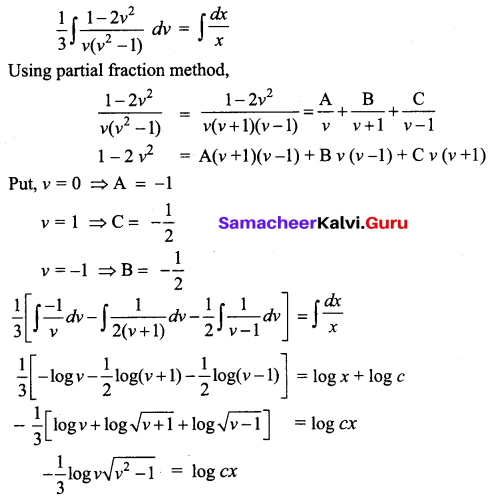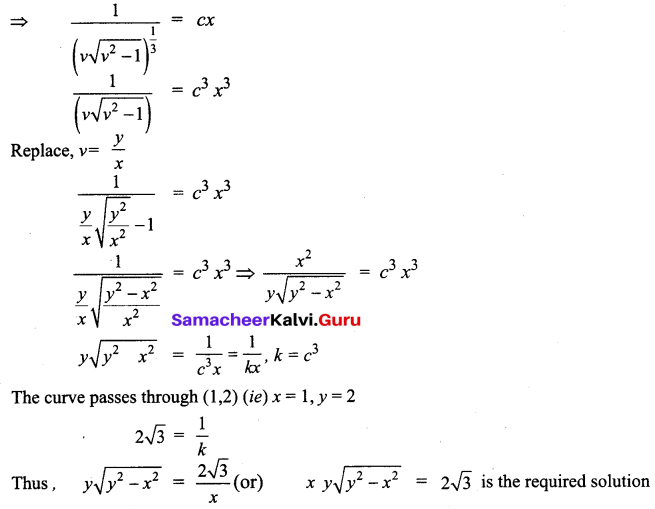Question 7.
An electric manufacturing company makes small household switches. The company estimates the marginal revenue function for these switches to be (x2 + y2) dy = xy dx where x represents the number of units (in thousands). What is the total revenue function?
Solution: